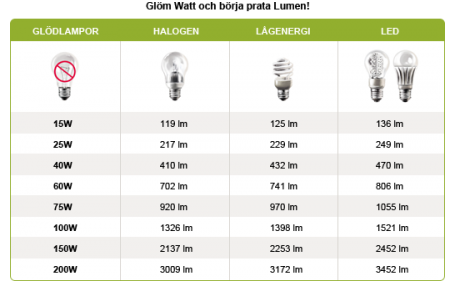Do you find it tricky to navigate all the different designations for luminous flux, luminous intensity etc.? Below you’ll find the answers to your questions. If you want to know more, you can contact our technical department at info@byrydens.se

What is lumen?
Answer: Lumen (lm) means luminous flux and is a measurement of how much light a specific light source emits in all directions.

What is candela?
Answer: Candela is the base unit of luminous intensity (brightness of a light) in a specific direction (angle). One candela is as much light as is emitted by a regular candle in a specific direction at a distance of one meter.

What is the difference between the above two terms?
Answer: Lumen is a measurement of how much light a light source produces and emits in all directions while candela is a measurement of the brightness of the light it emits in a specific angle.

What is luminous flux?
Answer: Luminous flux is the radiant intensity a light source emits in total in all directions. Its unit of measurement is lm (lumen).

What is luminous intensity?
Answer: Luminous intensity indicates the radiant intensity a light source or luminaire emits in a specific direction. If the angle is decreased, the brightness increases, and the other way around. The unit of measurement is cd (candela).

What is illuminance?
Answer: Illuminance is the luminous flux that reaches each square meter of a lit surface. Its unit of measurement is lux/m2. Illuminance is a quantitative concept that can easily be measured using a lux meter.

What is wattage?
Answer: Wattage indicates the power consumption of a light source, but says nothing about the amount of light it produces. It is indicated in W (watt).
Wattage (W) indicates the energy the light source uses per unit of time. For fluorescent lamps, other discharge lamps, low voltage halogen lamps and LEDs the ballast's self-consumption/energy waste is factored in. The total wattage is then referred to as system wattage.

What is luminous efficacy?
Answer: Luminous efficacy is a measurement of effectiveness and indicates how much light is produced for each watt used and provides a measurement for how economic the light source is. Indicated in lm/W (lumen/watt).

What is watt?
Answer: Wattage (W) indicates the energy the light source uses per unit of time. Watt equals the sum of Volts multiplied by Ampere.